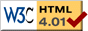# Pendulum with Direction Field

previous next
• Sim
• Graph
• Time Graph
• Multi Graph

Physics-based simulation of a pendulum, showing both a phase graph and the direction field (or slope field) associated with the differential equation.

Click the "Sim" tab to access parameters such as: gravity, mass or friction (damping). Drag the pendulum to change the starting position. Or click below to set some predefined initial conditions.

See the page about the single pendulum for more about how pendulums work and the math behind this simulation. This simulation is identical to that one, but adds a graph of the direction field (AKA slope field) of the differential equation. This direction field is shown overlapping the phase space graph of angular velocity vs. angle. At each point, the path of the pendulum in phase space is along the direction field.

Also available are: open source code, documentation and a simple-compiled version which is more customizable.

## Experiments to try:

• Notice that the path through phase space is always along the direction field.
• Which parameters (damping, gravity, mass, length) change the direction field? Why do they or don't they change the direction field?
• Try bigger faster motions of the pendulum (drag the pendulum to a high starting point, or give it a high start velocity). Notice that it still moves along the direction field in phase space.
• Turn on "show energy". When is kinetic energy highest? When is potential energy highest?
previous next